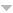## Publications

Export 25 results:
Sort by: Author [ Title] Type Year
Filters: First Letter Of Last Name is L  [Clear All Filters]
V
S
Journal of Functional Analysis, vol. 212, no. 1, pp. 89 - 120, 2004.
R
Ann. Probab., vol. 30, pp. 874- 897, 2002.
Q
Math. Nachrichten, vol. 183, pp. 113-133, 1997.
Journal of Mathematical Analysis and Applications, vol. 318, no. 2, pp. 507 - 516, 2006.
Math. Proc. Cambridge Phil. Soc., vol. 136, pp. 687-717, 2004.
Theory Probab. Appl., vol. 53, pp. 216-241, 2009.
Math. Nachrichten, vol. 241, pp. 103-109 , 2002.
P
Math. Proc. Cambridge Phil. Soc. , vol. 138, pp. 513-531, 2005.
O
J. Approx. Theory, vol. 144, no. 1, Orlando, FL, USA, Academic Press, Inc., pp. 27–53, 2007.
L
Information Theory, IEEE Transactions on, vol. 28, no. 2, pp. 129–137, 1982.
H
F
Annals of Applied Probability, vol. 18, pp. 427-469, 2008.
Journal of Functional Analysis, vol. 196, no. 2: Academic Press, pp. 486–531, December, 2002.
Stochastic Processes and their Applications, vol. 116, no. 2, pp. 310 - 336, 2006.
Journal of Theoretical Probability, vol. 16(4), 2003.
Mathematical Proceedings of the Cambridge Philosophical Society, 2010.
Foundations of quantization for probability distributions, , Secaucus, NJ, USA, Springer-Verlag New York, Inc., 2000.
E
Proceedings AMS, vol. 133, pp. 3403-3409 , 2005.
C
Journal of Applied Mathematics, vol. 2010, 2010.  Download: Constructive functional quantization.pdf (1.03 MB)
Mathematik: Universität Trier, 2008.
A
Real Analysis Exchange 26, vol. 26(2), pp. 795-810, 2001.
ESAIM: PS, vol. 14, pp. 93 - 116, 2010.